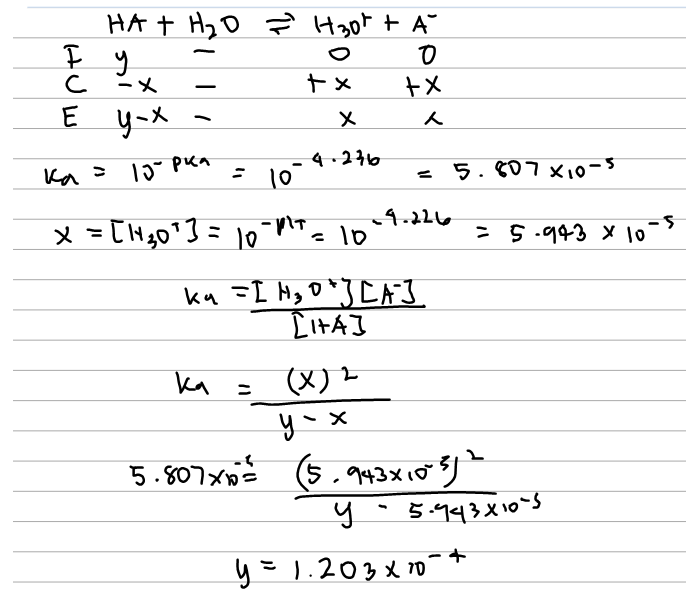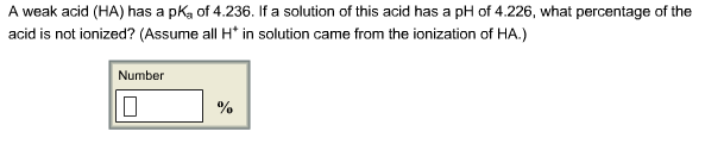# Problem: A weak acid (HA) has a pKa of 4.236. If a solution of this acid has a pH of 4.226, what percentage of the acid is not ionized? (Assume all H* in solution came from the ionization of HA.)

###### FREE Expert Solution82% (107 ratings)###### Problem Details

A weak acid (HA) has a pKa of 4.236. If a solution of this acid has a pH of 4.226, what percentage of the acid is not ionized? (Assume all H* in solution came from the ionization of HA.)